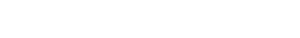# Fuel Cost Calculator

Welcome to fuel cost calculator, our website about calculating the fuel cost depending on the distance traveled, the fuel consumption or the fuel efficiency, and the fuel price. The fuel consumption expresses units of fuel per fixed distance, e.g. L/100km, whereas the fuel economy expresses units of distance per fixed fuel unit, e.g. MPG. The fuel cost is equal to the distance multiplied by the gas price, divided by the fuel efficiency or multiplied by the fuel consumption:

Fuel Cost = (Distance × Fuel Price) / Fuel Efficiency or Fuel Cost = (Distance × Fuel Price) × Fuel Consumption. We recommend using our fuel cost calculator right below:

0.00

Using our fuel cost calculator is straightforward, once all three entries have been made the result is displayed automatically. To start over press reset, or overwrite the numbers.

If you use our formulas above, then make sure to get the units right, something which our calculator does automatically for you, even if you mix English and metric units.

And if you are happy with our fuel cost calculator, then make sure to bookmark it now and to press the share buttons to let your friends know about our site and calculator.

## Trip Fuel Cost Calculator

Our trip fuel cost calculator above can be used with liters, kilometer, miles and gallons.

You can use it with the unit imperial gallon defined as 4.54609 liters or with the unit US liquid gallon of 3.785411784 liters, just be consistent when combining MPG with a fuel price in gallons.

Thus, if you have been looking for fuel cost calculator UK, then you have come to the right website, too. The same is true if you googled for trip fuel cost calculator for example.

Needless to say the currency does not enter the calculation, and that for round trips you have to double the distance; our fuel cost calculator does the math for one way by default.

Below you can find our search conversions form. With that form you can locate many fuel efficiency and fuel consumption conversions. Give it a try now!

 👉 🛒🎁 Our TOP PICKS For You 🤩💲👍

The conversions of units are based on these formulas:

• Miles per US gallon to L/100 km: 235.214583 / [US MPG] = [L/100km]
• Miles per UK gallon to L/100 km: 282.481053 / [UK MPG] = [L/100km]
• L/100km to Miles per US gallon: 235.214583 / [L/100km] = [US MPG]
• L/100km to Miles per UK gallon: 282.481053 / [L/100km] = [UK MPG]
• Miles per US gallon to Km/L: [US MPG] / 235.214583 = [Km/L]
• Miles per UK gallon to Km/L: [UK MPG] / 282.481053 = [Km/L]
• Km/L to Miles per US gallon: [[Km/L] * 235.214583 = [US MPG]
• Km/L to Miles per UK gallon: [Km/L] * 282.481053 = [UK MPG]

For precise conversions you have to use 235.214583 instead of 235, and in place of 282 use 282.48093633182215859381213711922; for Km/L you have to divide these numbers by 100.

You have reached the end of fuel cost calculator. This image sums it all up:Our frequent calculations include:

Submitting...